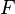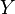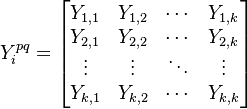# Final Demand

## Definition

Final Demand or final consumption in the context of an Input-Output Model denotes the ultimate destination of goods and services used up by individual households or the community to satisfy their individual or collective needs or wants.

## Formula

Final demand might be denoted as a vector or matrix (typically using$F$ or$Y$).

### Single Region Case

In the single region case final demand is a vector and depicted as a column next to the Input-Output Matrix.

### Multi-Region Case

In the multi-region case, the global final demand a tensor, usually represented as a matrix of vectors and denoted as "Y":\begin{align} Y^{pq}_{i} = \begin{bmatrix} Y_{1,1} & Y_{1,2} & \cdots & Y_{1,k} \\ Y_{2,1} & Y_{2,2} & \cdots & Y_{2,k} \\ \vdots & \vdots & \ddots & \vdots \\ Y_{k,1} & Y_{k,2} & \cdots & Y_{k,k} \end{bmatrix} \end{align}

with the final demand vector of domestic (country q) production in the main diagonal and direct imports to final demand from country p captured by off-diagonal vectors.

## Usage

The precise nature of final demand depends on the economic system modeled and may include:

Adjusting final demand is and exploring economic implications is a core element of Input-Output Analysis.